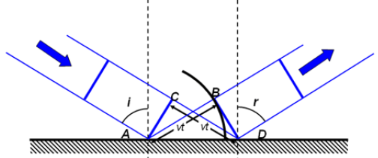### Reflection:

Each point on a plane wavefront can be considered to be a circular ripple. The time taken for the ripple at A to get to B is the same as the time taken for the ripple at C to get to D. the speed is unchanged in the medium, so simple geometry shows that the angle of incidence equals the angle of reflection.### Refraction:

Each point of contact of a wavefront along the boundary between media of different refractive indices n (m) becomes a point-source emitter of waves whose velocity depends on n (m). The tangents of the circles representing the point-source emission define the refraction angles.The Snell’s law is also proved by the Huygens principle. This shows the speed of light in water is less than the speed in air.

This experiment was found correct by Foucault. If the refraction is occurring at the rarer medium and angle of incidence is greater than the critical angle, then this is the case for total internal reflection to occur.